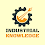## What is a Scatter Diagram in 7 QC Tools?

→ Scatter Diagram is used to study and identify the possible relationship between two variables.
→ It is also used to validate the relationship between cause and effect and it is also known as the validation tool.
→ Scatter Chart in 7 QC Tools is a graph in which the values of two variables are plotted along two axes of the graph, the pattern of the resulting points will say the correlation.
→ We use this chart to find out the relation between cause and effect by using a cause and effect diagram.
→ This tool is commonly used in the analyze phase in the Six Sigma Methodology.

### Scatter Plot Examples

→ Refer below to the relations of two variables that we can find in our real life.
• Weight and Height of a Man.
• Hardness and carbon content in the product.
• Visual Inspection mistakes and Illumination levels.
• Child’s height and Father’s height.
• Curing Temperature and Curing Time.

### What is a scatter diagram used for?

• It is used for the validation of two different variable
• It is also used for checking the trend with respect to time
• The Scatter_diagram is used to confirm a hypothesis testing between two variables.

## How to draw a Scatter Diagram?

→  Refer to the four steps for Making a Scatter_Graph.
1. Data Collection
2. Choose Independent and Dependent variables.
3. Construct the Graph and add the titles & trend line.
4. Interpret the Graph

#### Step 1. Data Collection:

→ Now we are taking one example to understand how to make a chart?
→ In this example, we are taking 50 readings of different curing temperatures on different curing times for a product manufactured on the thermosetting press. we want to find out the relationship between curing time and curing temperature.
→ We have to find if any correlation is present or not between curing temperature and curing time.
→ If we have more data samples then it will give a more precise result.

#### Step 2. Choose Independent and Dependent Variables:

→ The dependent variable is usually plotted along the vertical axis i.e. in Y-axis and it is called a measured parameter.
→ The independent variable is usually plotted along the horizontal axis i.e. in X-axis and it is called a control parameter.
→ In this case, we are taking heating temperature as an independent variable on the x-axis, and curing time is dependent on heating temp. so we mentioned it on the y-axis.

#### Step 3. Construct the Graph and add the titles & trend line:

→ Now based on recorded data construct a graph and add a suitable title, horizontal axis name, vertical axis name, and make trend line.

#### Step 4. Interpret the Graph

→ We will interpret the chart based on the trend line.

### Types of Correlation in Scatter Diagram in 7 QC Tools:

→ There are many different types of correlation found between the Independent and Dependent variables which are mentioned below with pictorial representation.
→ Mainly three relations available between two variables we can say that Strong, Moderate, and No Relation.
→ A strong positive correlation means it is a clearly visible upward trend from left to right, a strong negative correlation means it is a clearly visible downward trend from left to right.
→ eg. In positive relation, as the value of x increases, the value of y will also increase we can say that the slope of the straight line drawn along the data points will go up and the pattern will resemble the straight line.
→ For example, in the summer season the temperature increase, icecream sales will also increase.
→ A negative correlation, as the value of x increases, the value of y will decrease and the slope of a straight line drawn along the data points will go down.
→ For example, in the summer season the temperature increase, the sales of winter coats decrease.
→ A weak correlation means it is less clear that the relationship is either positive or negative?
→ No correlation means neither positive nor negative relation and indicates the independent variable does not affect the dependent variable.

### What are the 3 types of scatter plots?

→ There are main three types mentioned below.
1. Positive
2. Negative
3. Neutral
→ The subtypes of the scatter_plot are mentioned below and you can refer the pictures for your better understanding.
⇢ (1) Strong Positive
⇢ (2) Moderate Positive
⇢ (3) Weak Positive

⇢ (4). Strong Negative
⇢ (5). Moderate Negative
⇢ (6). Weak Negative
⇢ (7). Random Pattern

### Benefits of Scatter Diagram:

→ It is beneficial to confirm a hypothesis (assumption) between two variables that are related or not.
→ Provide both visual and statistical means to test the strength of a potential relationship.
→ It is a very good validation tool.
→ Used for proving the relation between cause and effect.
→ Plotting the diagram is relatively simple.

### Limitation of Scatter Plot:

→ It does not show you the quantitative measure of the relationship between the variable.
→ This chart does not show you the relationship for more than two variables at a time.

1.Great presentation

1.Thank you for your kind word

2.you know , you are amazing

1.3.Very good information for us

1.4.1.